# OECD Nuclear power plants data analysis 3 - Hypothesis testing using R with infer packageUnsplashYan Agritが撮影した写真

This post is following of the above post.

In this post I do hypothesis testing using R with infer package.

Let's go.

Firstly I set Null hypothesis and Alternative hypothesis as follows.

Null: avaerage number of nuclear power plant is 31.

Alternative: average number of nuclear power plant is less than 31.

And I set alpha = 0.5%.

So, Null: mu = 31, Alternative: mu < 31, alpha = 0.05.

All right, let's use R.

I make null distribution.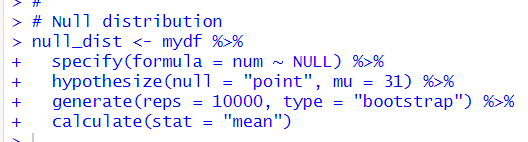Then, I visualize this distribution.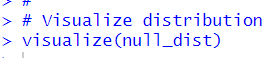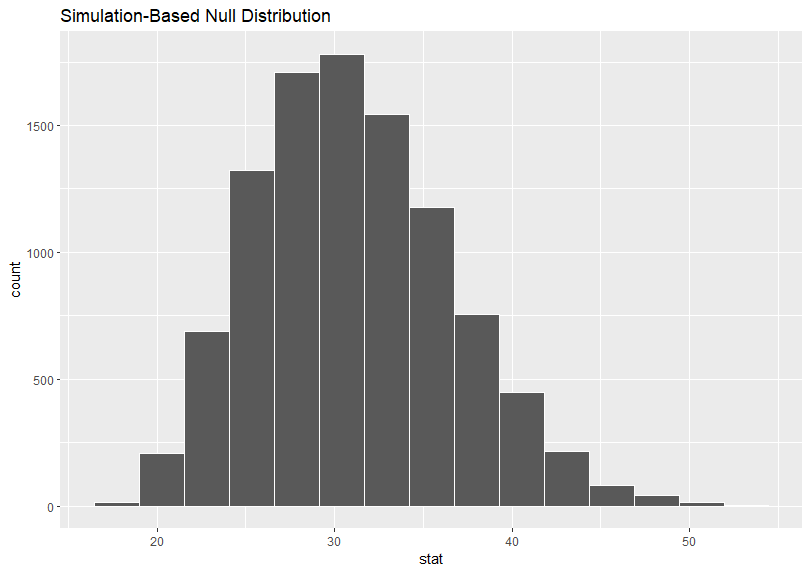To comapre this distribution with observed average, I calculate observed average.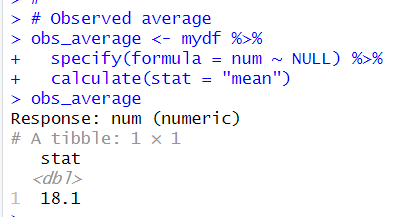Then, let's see our p-value.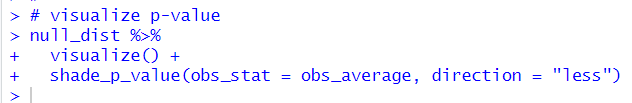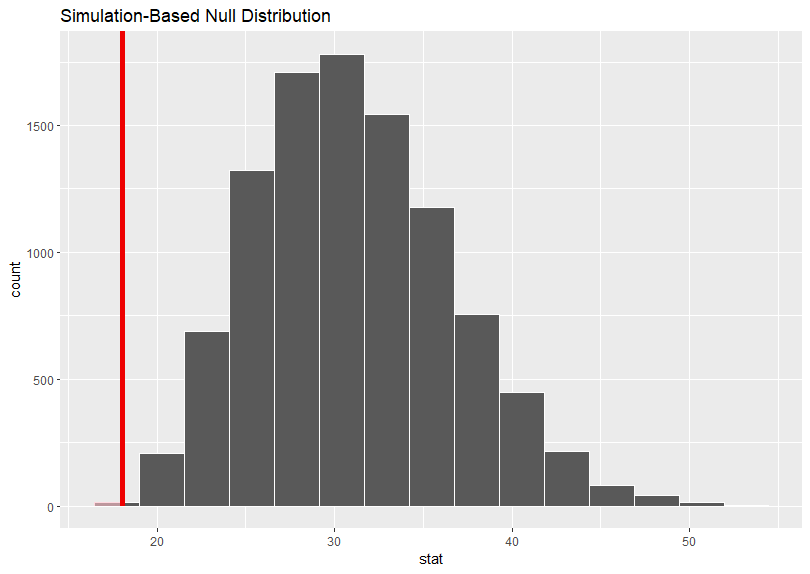The red vertical line is observed average line.

Let's calculate p-value.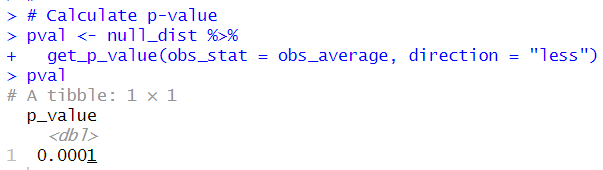p-value is 0.001 and I set alpha = 0.05, so I can reject Null hypothesis: average number of nuclear power plants is 31 at the 5% level..

Our sample size is only 19, so I cannot use traditional(formula base) hypothesis testing.

And Q-Q plot is not showing normal distribution.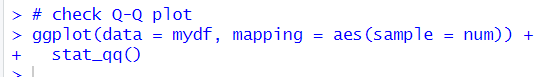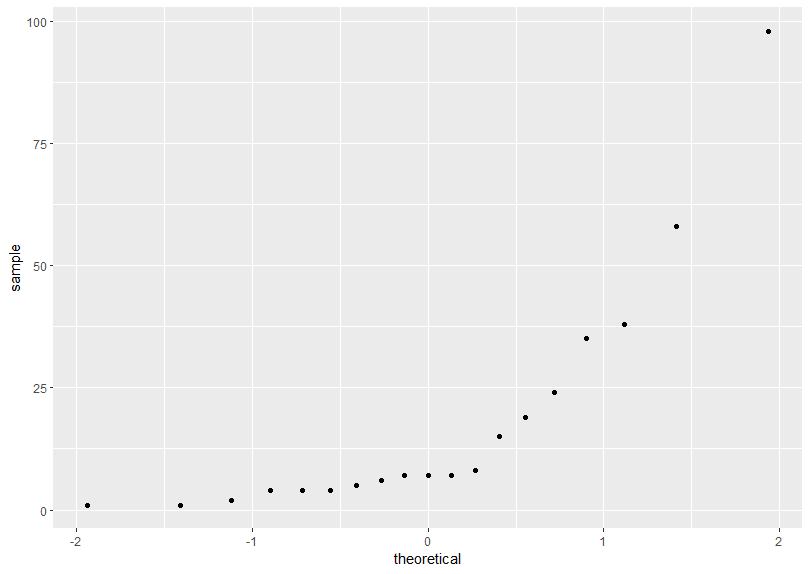Anyway, I just use t.test() function and t_test() function to do traditional(formula based) hypothesis testing.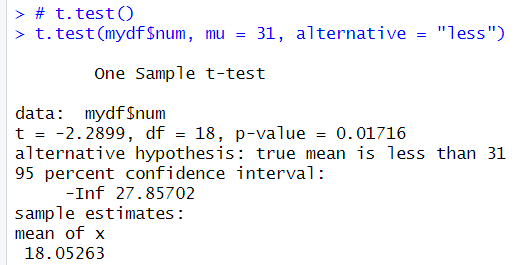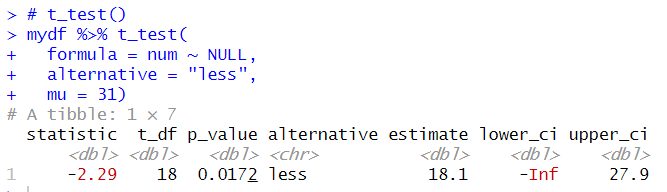The both t.test()and t_test() returns same p-value, 0.0172, it is greater than bootstrapped p-value: 0.0001.
So, I am more confident with bootstrapping hypothesis testing in this case.
That's it Thank you!

Next post is

To read from the 1st post,